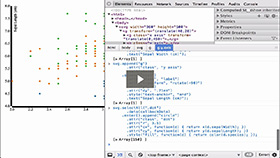Basic Chart - Scatterplot
Duration: 22:46
Part of Course: Introductory D3 Course
This Lesson is for subscribersUnlock this lesson NOW!
• Get expert advice on Basic Chart - Scatterplot
• Start mastering D3.js right now!

Takeaways:

• You will use the TSV data from the D3js.org website Scatterplot Example to see how a full D3 Scatterplot data visualization is built
• Notice that the styling is done in the <style> </style> section of the HTML document
• Notice the D3 Margin Convention
• Notice the D3 Linear Scale for the X-Axis
• Notice the D3 Linear Scale for the Y-Axis
• Notice the D3 Ordinal Scale for color - d3.scale.category10()
• Notice the D3 SVG Axis Component creation and definition of the X-Axis and Y-Axis
• Notice the D3 Type-specific XHR call - d3.tsv(...)
• Notice the setting of the domain for the X-Axis and Y-Axis scales
• Notice the use of the D3 .nice() functionality
• Notice the D3 SVG Axis Component instantiation of the X-Axis and Y-Axis
• Notice the D3 Data Join
• Notice how D3 helps create visual representations of the data
• Notice how a second D3 Data Join is used to create the legend for the Scatterplot Visualization

Resources:
This Transcript is for subscribers.Unlock this D3 Tutorial NOW!
• Get expert advice on Basic Chart - Scatterplot